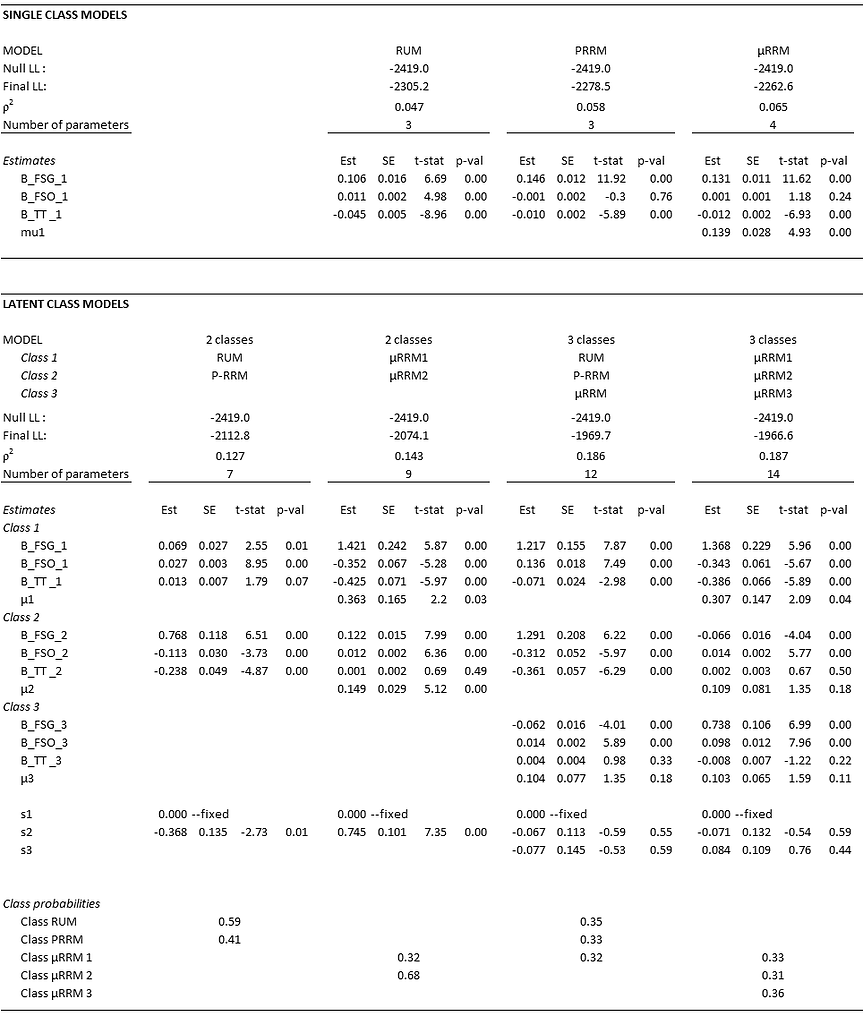## Latent Class models

Latent class (LC) models are increasingly used in choice analysis, and are particularly suitable to investigate the existence of decision rule heterogeneity.

In the LC model the probability that decision maker n chooses alternative i, equals the sum of the probability that he/she belongs to class s multiplied by the probability that i is chosen given the class s.

The class membership model π_ns is typically a logit model. Class membership is a function f (*) of explanatory variables z_n (e.g. socio-demographic characteristics), where η_s denotes a vector of class-membership parameters that need to be estimated, and δ_s denotes class-specific constants.

In the context of advanced RRM models, an interesting research avenue is to define classes corresponding to different decision models.

Below three LC applications are given:

1) a two-class model comprising of a RUM class and a P-RRM class                                    (PYTHON, PANDAS, Apollo R and MATLAB).

2) a two-class model comprising of two μRRM classes                                                           (PYTHON, PANDAS, LatentGOLD, Apollo and MATLAB).

3) a three-class model comprising of a RUM class, a P-RRM class and a μRRM class        (PYTHON, PANDAS, Apollo R and MATLAB).

Since in the shopping choice data do not contain explanatory variables that can be used to explain class membership, only class-specific constants are estimated (hence, the LC models are basically discrete mixture models)

Software code to estimate LC models is available for BIOGEME (PYTHON & PANDAS), Apollo RLatentGOLD CHOICEand MATLAB. Because of the ease of interpretation, the MATLAB code uses Maximum Likelihood Estimation (MLE). However, MLE is relatively slow for Latent Class discrete choice models. Estimation code based on Expectation-Maximisation is distributed on request. Furthermore, note that the parameterisation of the μRRM model in LatentGOLD CHOICE is somewhat different from the parameterisation in Cranenburgh et al. 2015. Therefore, an accompanying document is provided showing how to compare the results of LatentGOLD with e.g. MATLAB.

MATLAB

• Click here for a bundle of MATLAB codes to estimate Latent class models.

PYTHON BIOGEME

• Click here for PYTHON BIOGEME code to estimate a two-class model comprising of a RUM and a P-RRM class.

• Click here for PYTHON BIOGEME code to estimate a two-class model comprising of two μRRM classes.

• Click here for PYTHON BIOGEME code to estimate a three-class model comprising of a RUM class, a P-RRM class and a μRRM class

PANDAS BIOGEME

• Click here for PANDAS BIOGEME code to estimate a two-class model comprising of a RUM and a P-RRM class.

• Click here for PANDAS BIOGEME code to estimate a two-class model comprising of two μRRM classes.

• Click here for PANDAS BIOGEME code to estimate a three-class model comprising of a RUM class, a P-RRM class and a μRRM class

Apollo R

• Click here for Apollo R code to estimate a two-class model comprising of a RUM and a P-RRM class.

• Click here for Apollo R code to estimate a two-class model comprising of two μRRM classes.

• Click here for Apollo R code to estimate a three-class model comprising of a RUM class, a P-RRM class and a μRRM class

LatentGOLD

• Click here for a bundle of LatentGOLD codes to estimate Latent class RRM models.

EXAMPLE DATA FILE